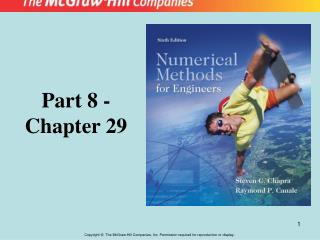DownloadDownload PresentationPart 8 - Chapter 29

# Part 8 - Chapter 29

Télécharger la présentation## Part 8 - Chapter 29

- - - - - - - - - - - - - - - - - - - - - - - - - - - E N D - - - - - - - - - - - - - - - - - - - - - - - - - - -
##### Presentation Transcript

1. Part 8 - Chapter 29

2. Part 8Partial Differential Equations Table PT8.1

3. Figure PT8.4

4. Finite Difference: Elliptic EquationsChapter 29 Solution Technique • Elliptic equations in engineering are typically used to characterize steady-state, boundary value problems. • For numerical solution of elliptic PDEs, the PDE is transformed into an algebraic difference equation. • Because of its simplicity and general relevance to most areas of engineering, we will use a heated plate as an example for solving elliptic PDEs.

5. Figure 29.1

6. Figure 29.3

7. The Laplacian Difference Equations/ Laplace Equation O[D(x)2] O[D(y)2] Laplacian difference equation. Holds for all interior points

8. Figure 29.4

9. In addition, boundary conditions along the edges must be specified to obtain a unique solution. • The simplest case is where the temperature at the boundary is set at a fixed value, Dirichlet boundary condition. • A balance for node (1,1) is: • Similar equations can be developed for other interior points to result a set of simultaneous equations.

10. The result is a set of nine simultaneous equations with nine unknowns:

11. The Liebmann Method/ • Most numerical solutions of Laplace equation involve systems that are very large. • For larger size grids, a significant number of terms will b e zero. • For such sparse systems, most commonly employed approach is Gauss-Seidel, which when applied to PDEs is also referred as Liebmann’s method.

12. Boundary Conditions • We will address problems that involve boundaries at which the derivative is specified and boundaries that are irregularly shaped. Derivative Boundary Conditions/ • Known as a Neumann boundary condition. • For the heated plate problem, heat flux is specified at the boundary, rather than the temperature. • If the edge is insulated, this derivative becomes zero.

13. Figure 29.7

14. Thus, the derivative has been incorporated into the balance. • Similar relationships can be developed for derivative boundary conditions at the other edges.

15. Figure 29.9 Irregular Boundaries • Many engineering problems exhibit irregular boundaries.

16. First derivatives in the x direction can be approximated as:

17. Figure 29.12 • A similar equation can be developed in the y direction. Control-Volume Approach

18. Figure 29.13

19. The control-volume approach resembles the point-wise approach in that points are determined across the domain. • In this case, rather than approximating the PDE at a point, the approximation is applied to a volume surrounding the point.